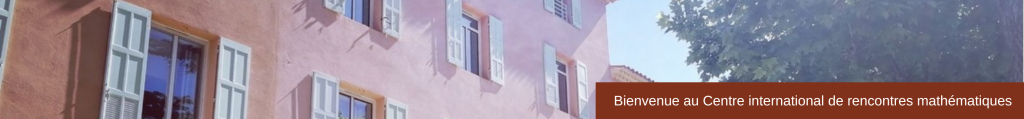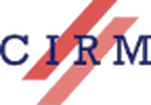CONFERENCE
Evolution Equations on Singular Spaces
April 25-29, 2016
 The meeting will focus on the behavior of waves in the presence of singular structures, ranging from boundaries to corners, edges, cones, and cusps. Among other subtleties introduced into the propagation of wavefronts, the phenomenon of diffraction is of central importance and has considerable mathematical interest. The meeting will bring together researchers from a number of areas, including both elliptic and hyperbolic partial differential equations as well as applied mathematics and numerical analysis. ﻿Scientific & Organizing Committee Dean Baskin (Texas A&M University)Luc Hillairet (Université d’Orléans)Jared Wunsch (Northwestern University) Speakers Matthew Blair﻿ (University of New Mexico) Lp bounds on eigenfunctions of the Laplacian on polygonal domains Virginie Bonnaillie-Noël (ENS Paris) Magnetic Laplacian in singular domains Nicolas Burq (Université Paris-Sud) Decay for the damped wave equation in unbounded domains Simon Chandler-Wilde (University of Reading) Hybrid Asymptotic-Numerical Integral Equation Methods forHigh Frequency Scattering – Part. 1 Jeffrey Galkowski (Stanford University) A Quantum Sabine Law for Resonances in Transmission Problems Jesse Gell-Redman (Johns Hopkins University) The Feynman Propagator on Asymptotically Minkowski Spaces. Colin Guillarmou (ENS Paris) Correspondence between Ruelle resonances and quantum resonances fornon-compact Riemann surfaces Peter Hintz (University of California, Berkeley) Regularity of waves at the Cauchy horizon of black hole spacetimes Oana Ivanovici (Université Nice Sophia Antipolis) Dispersion estimates for the wave and the Schrodinger equations out-side strictly convex obstacle Christopher Judge (Indiana University) Triangles in the hyperbolic plane with no positive Neumann eigenvalues Gilles Lebeau (Université Nice Sophia Antipolis) On the holomorphic extension of the Poisson Kernel Anna Mazzucato (Penn State University) Mixed-boundary-value and transmission problems on generalized polyhedral domains Richard Melrose (MIT) The wave equation on Weil-Petersson spaces Nicolas Popoff (Université Bordeaux 1) Ground state energy of the Robin Laplacian in corner domains Julie Rowlett (Chalmers Technical University) A Polyakov formula for angular variations Elmar Schrohe (University of Hannover) The porous medium equation on manifolds with conical singularities Euan Spence (University of Bath) Hybrid Asymptotic-Numerical Integral Equation Methods for High Fre-quency Scattering – Part. 2 Andras Vasy (Stanford University) Asymptotics of radiation fields on long-range asymptotically Minkowski spaces Boris Vertman (Universities of Bonn and Münster) Ricci Flow on singular edge manifolds Goergi Vodev (Université de Nantes) Asymptotic behavior of the interior transmission eigenvalues